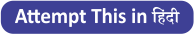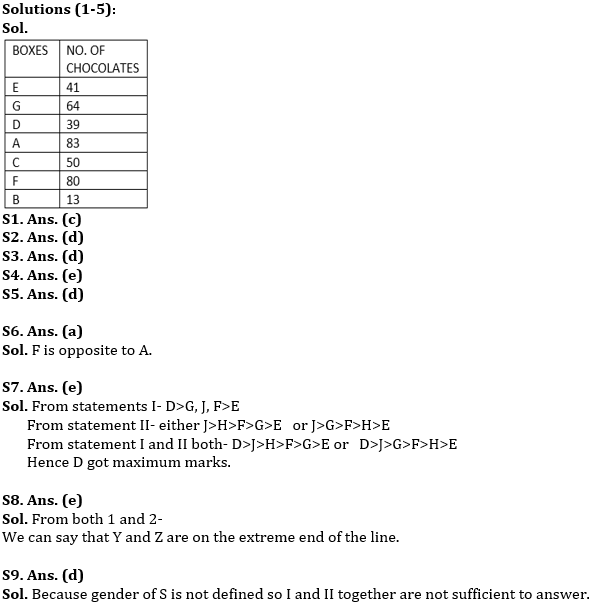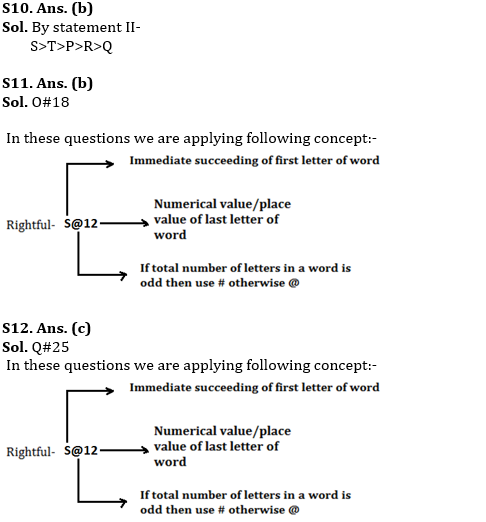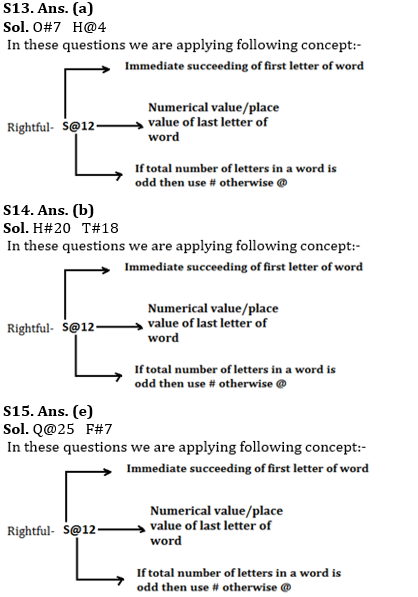Latest Banking jobs   »

# Reasoning Ability Quiz For RBI Assistant/ ESIC UDC Mains 2022- 16th April

Directions (1-5): Study the following information carefully and answer the questions given below:
Seven boxes A, B, C, D, E, F and G are kept one above the other containing different number of chocolates. Not more than three boxes are kept below A. Two boxes are kept between A and the box containing 41 chocolates which is kept above Box A. D contains thrice the number of chocolates than box B. Box C contains 50 number of chocolates. The number of chocolates in box G is a cube of a number. Only one box is kept between box containing 41 chocolates and the box which contains 39 chocolates. Only one box is between the box containing 50 chocolates and the one which contains 39 chocolates. Two boxes are between the box containing 50 chocolates and the one which contains 64 chocolates. The number of boxes between C and D is same as the number of boxes between C and the box which contains 13 chocolates. Box F contains 80 chocolates. One box contains 83 chocolates.Q1. How many box/boxes is/are kept between Box D and Box B?
(a) one
(b) two
(c) three
(d) more than three
(e) none

Q2. How many chocolates are kept in box E?
(a) 50
(b) 13
(c) 83
(d) 41
(e) none of these

Q3. Which among the following boxes contains the maximum and minimum number of chocolates respectively?
(a) G, E
(b) B, D
(c) B, A
(d) A, B
(e) none of these

Q4. Which of the following combination is not true?
(a) 50-C
(b) 13-B
(c) 41-E
(d) 64-G
(e) All are true

Q5. Which among the following boxes is kept immediately below box B?
(a) G
(b) C
(c) A
(d) No One
(e) none of these

Directions (6-10): Each of the following questions below consists of a question and two statements numbered I and II given below it. You have to decide whether the data provided in the statements are sufficient to answer the question. Read both the statements and give answer.

Q6. Eight friends A, B, C, D, E, F, G and H are sitting around a circular table facing the center. Who is opposite to A?
I. D sits second to right of F and opposite to E. A is an immediate neighbor of B and C.
II. G sits third to right of E, who sits second to right of A.
(a) if the data in statement I alone are sufficient to answer the question, while the data in statement II alone are not sufficient in answer the question.
(b) if the data in statement II alone are sufficient to answer the question, while the data in statement I alone are not sufficient to answer the question.
(c) if the data in either in statement I alone or in statement II alone are sufficient to answer the question.
(d) if the data in both the statements I and II together are not sufficient to answer the question.
(e) if the data in both the statements I and II are together necessary to answer the question.

Q7. Who among D, E, F, G, H and J got the highest marks in an exam?
I. D’s marks was more than G and J and E’s marks was less than that of F.
II. E scored less than G. J scored more than G and H. No one scored marks between F and G or F and H.
(a) if the data in statement I alone are sufficient to answer the question, while the data in statement II alone are not sufficient in answer the question.
(b) if the data in statement II alone are sufficient to answer the question, while the data in statement I alone are not sufficient to answer the question.
(c) if the data in either in statement I alone or in statement II alone are sufficient to answer the question.
(d) if the data in both the statements I and II together are not sufficient to answer the question.
(e) if the data in both the statements I and II are together necessary to answer the question.

Q8. Among V, W, X, Y and Z, seated in a straight-line facing south, who sits at the extreme end of the row?
I. W sits second to right of V. Z is not immediate neighbor of Y.
II. V sits third to left of Y. W sits on the immediate right of X.
(a) if the data in statement I alone are sufficient to answer the question, while the data in statement II alone are not sufficient in answer the question.
(b) if the data in statement II alone are sufficient to answer the question, while the data in statement I alone are not sufficient to answer the question.
(c) if the data in either in statement I alone or in statement II alone are sufficient to answer the question.
(d) if the data in both the statements I and II together are not sufficient to answer the question.
(e) if the data in both the statements I and II are together necessary to answer the question.

Q9. How is S related to E ?
I. W is father of X and P is grandson of E, who is wife of W.
II. G is mother of S and daughter in law of W.
(a) if the data in statement I alone are sufficient to answer the question, while the data in statement II alone are not sufficient in answer the question.
(b) if the data in statement II alone are sufficient to answer the question, while the data in statement I alone are not sufficient to answer the question.
(c) if the data in either in statement I alone or in statement II alone are sufficient to answer the question.
(d) if the data in both the statements I and II together are not sufficient to answer the question.
(e) if the data in both the statements I and II are together necessary to answer the question.

Q10. Among five friends P, Q, R, S and T having different heights, who is the tallest?
I. R is taller than only one friend. Only one friend is taller than T. P is not shortest.
II. R is shorter than three persons. Only one person is taller than T. P is neither the tallest nor the shortest in the group. Q is the shortest in group.
(a) if the data in statement I alone are sufficient to answer the question, while the data in statement II alone are not sufficient in answer the question.
(b) if the data in statement II alone are sufficient to answer the question, while the data in statement I alone are not sufficient to answer the question.
(c) if the data in either in statement I alone or in statement II alone are sufficient to answer the question.
(d) if the data in both the statements I and II together are not sufficient to answer the question.
(e) if the data in both the statements I and II are together necessary to answer the question.

Directions (11-15): Study the information and answer the following questions:
In a certain code language
“Rightful due political economy” is coded as ” S@12 E#5 Q#12 F#25″
“There are numerous ways ” is coded as ” U#5 B#5 O@19 X@19″
“Work culture provide good” is coded as ” X@11 D#5 Q#5 H@4″

Q11. What is the code for ‘neighbour’ in the given code language?
(a) M#20
(b) O#18
(c) N#18
(d) S#20
(e)None of these

Q12. What is the code for ‘proximity’ in the given code language?
(a) R#25
(b) M#25
(c) Q#25
(d) L#25
(e)None of these

Q13. What may be the possible code for ‘Nothing good’ in the given code language?
(a) O#7 H@4
(b) S@5 X#12
(c) O@5 H#15
(d) T@5 W#12
(e)None of these

Q14. What may be the possible code for ‘great scholar’ in the given code language?
(a) X#7 V@5
(b) H#20 T#18
(c) H@7 T@5
(d) G#7 T@5
(e)None of these

Q15. What is the code for ‘publicly enforcing’ in the given code language?
(a) R@25 F#7
(b) Q#25 F#7
(c) Q@25 F@7
(d) Q@12 F#14
(e)None of these

Solutions